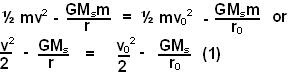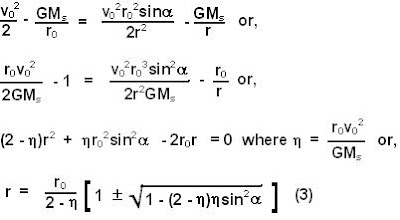## Tuesday, September 9, 2008

### Irodov Problem 1.209The problem can be solved using the two basic conservation principles, i) conservation of angular momentum and ii) conservation of energy.

Let r be the distance of closest/farthest approach of the planet to the sun and v be its tangential velocity at this point. So the sum of the planet's kinetic energy and its potential energy must be conserved at all times. In other words,Here m is the mass of the planet and Ms the mass of the sun.

The angular momentum of the planet is given by m v x r. Since angular momentum must be conserved, the planet's angular momentum at the closest/farthest approach will be exactly equal to its angular momentum when it is located at r0. In other words,From (1) and (2) we have,(3) has two solutions corresponding to the farthest (+ sign) and nearest (- sign) approaches in the planet.